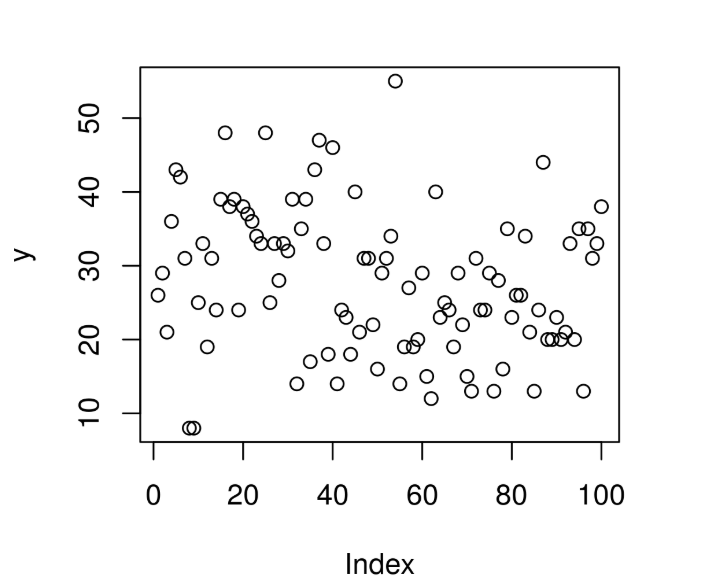Related Articles
Compute Randomly Drawn Wilcoxon Signedrank Density in R Programming – rsignrank() Function
• Last Updated : 25 Jun, 2020

`rsignrank()` function in R Language is used to compute random density for Wilcoxon Signedrank Statistic Distribution over a sequence of random Numeric values.

Syntax: rsignrank(N, n)

Parameters:
N: Size of Random Vector
n: Sample Size

Example 1:

 `# R Program to compute random``# Wilcoxon Signedrank Density`` ` `# Setting seed for``# random number generation``set``.seed(``1000``)`` ` `# Set Vector size``N <``-` `20`` ` `# Calling rsignrank() Function``y <``-` `rsignrank(N, n ``=` `10``)``y`

Output:

```  26 29 21 36 43 42 31  8  8 25 33 19 31 24 39 48 38 39 24 38
```

Example 2:

 `# R Program to compute random``# Wilcoxon Signedrank Density`` ` `# Setting seed for``# random number generation``set``.seed(``1000``)`` ` `# Set Vector size``N <``-` `100`` ` `# Calling rsignrank() Function``y <``-` `rsignrank(N, n ``=` `10``)`` ` `# Plot a graph``plot(y)`

Output:Attention reader! Don’t stop learning now. Get hold of all the important DSA concepts with the DSA Self Paced Course at a student-friendly price and become industry ready.

My Personal Notes arrow_drop_up